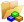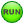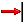# MV-2051: Frequency Response Analysis using MotionSolve and Compose

In this tutorial, you will learn how to convert a multibody model to its equivalent state space form using MotionSolve and obtain corresponding matrices, use these state space matrices to construct an equivalent LTI system of the multibody model within Altair’s Compose, and perform a frequency response analysis on the LTI system by plotting a Bode Plot diagram.

This exercise uses the SimplifiedTwoSpring_LTI_START.mdl file located in the mv_hv_hg\mbd_modeling\linear folder.

This is an extension of tutorial - MV-2050: Linear Analysis for Stability and Vibration Analysis.

Control system design becomes computationally simpler using mathematical tools like transfer function that can only be applied on Linear time-invariant systems (LTI systems). Linear systems have outputs as a linear combination of inputs. A wide range of physical systems can be approximated very accurately by LTI models.

A Linear Time Invariant (LTI) system in the state space form is described as follows:where A, B, C, and D are the state matrices, x is the state vector, u is the input vector, and y is the output vector.

MotionSolve can linearize a multibody system by calculating the state matrices for a given set of inputs and outputs through a linear analysis. The states are chosen automatically by MotionSolve.

Frequency response concepts and techniques play an important role in control system design and analysis. In frequency-response methods, we vary the frequency of the input signal over a certain range and study the resulting response.

In this tutorial, you will learn how to convert a multibody model to its equivalent state space form using MotionSolve and obtain corresponding A, B, C and D matrices. These state space matrices will be further used to construct an equivalent LTI system of the multibody model within Altair’s Compose. Next, a frequency response analysis will be performed on the LTI system using Compose by plotting a Bode Plot diagram.

Next, you can then perform control system design using a variety of tools available within Compose, however this is out of the scope of this tutorial.

## Open and Review the Model

1. Open a new session of MotionView.
2. Click on Open Model,, from the Standard toolbar.
3. From the Open Model dialog, locate and select the SimplifiedTwoSpring_LTI_START.mdl file from your <working directory>.
4. Review the simplified model.
The Model contains:
1. Two bodies that represent the suspension and bus body.
2. Two springs connecting the bus, suspension and the road.
Now, consider a hypothetical force (obtained from a controller) that acts on suspension mass in order to improve the response of system (in other words, bus body displacement) under the effect of disturbance from road. A simplified block diagram of such a system showing its input and output is shown below.
5. The model contains following analyses:
1. Transient analysis consisting of a force and a motion.
2. Linear analysis consisting of a motion.

In the next step you will perform a transient analysis to identify the output of plant corresponding to a unit value of input.

## Transient Analysis - Response at First Mode

In previous tutorial (MV-2050) the first mode of this system was identified at 1.63Hz (~10.24 rad/s). In this step you will identify the response of this system (in other words, Displacement of Bus) when a unit force is applied at a forcing frequency of 10.24rad/s at Suspension Body.

1. Review the force.
2. Review the displacement motion (external disturbance to the plant) at Road, which is currently locked.
3. Run the model.
1. Go to the Run panel.
2. Rename the XML file to SimplifiedTwoSpring_Trans.xml.
3. Set Simulation type to Static+Linear.
4. Set Analysis to Transient_At_FirstMode.
4. Review the results in HyperGraph and plot the displacement output of BusBody in the vertical direction (Z axis).
In the next step you will perform a linear analysis to obtain a state space matrix that will be further used to construct an equivalent LTI system of this system.

## Linear Analysis - Plant Input

In this step you will setup the input of the plant (u). A control force will be applied on the Suspension Mass.

1. Activate analysis Linear Analysis in the model by right-clicking on Linear in the browser and then selecting Activate > Selected only.
Note: Review the existing motion in the analysis. An external disturbance is modelled as displacement motion at Road which is currently locked (in other words, zero displacement) to avoid transient conditions.
2. Add a SolverVariable to the analysis Linear Analysis that can obtain the input from the controller to the plant.
1. From the Project Browser, right-click Linear analysis and select Add > Control Entity > SolverVariable (or right-clickon the Control Entity toolbar after selecting Linear analysis in the Project Browser).
The Add SolverVariable dialog is displayed.
2. Change the Label to FromController_SuspForce. Leave the definition as the default.
3. Add a Solver Array to the analysis Linear Analysis to define input to the plant.
1. From the Project Browser, right-click Linear analysis and select Add > Control Entity > SolverArray (or right-clickon the Control Entity toolbar after selecting Linear analysis in Project Browser).
The Add SolverArray dialog is displayed.
2. Change the Label to Plant_Input and click OK.
3. From the panel, change Array type to Plant Input using the drop-down menu.
4. Choose the SolverVariable as FromController_SuspForce using the SolverVariable collector.
5. Verify that the Use in linearization check box is selected.
1. From the Project Browser, right-click Linear analysis and select Add > Force Entity > Force (or right-clickon the Force Entity toolbar after selecting Linear analysis in Project Browser).

The Add Force dialog is displayed.

2. Change the Label to ActiveSuspension_ControlForce and click OK.
3. From the panel, choose SuspensionMass as the Body.
Note: Double click on the Body collector to invoke the advanced entity selector dialog and turn off the Only show entities within valid scope option to be able to choose the SuspensionMass body which is outside the Analysis.
4. Choose SuspensionMassCG as the point of application of the force.
5. In the Tran Properties tab, change Fz to Expression and set it to {sv_fromcontroller_suspforce.VARVAL}.
Note: The Expression Builder can be used to create this expression.
The input from the controller as defined by the solver variable will now be used by the force entity as described by the expression.

## Linear Analysis - Plant Output

In this step you will set up the output of the plant (y). A solver variable and solver array will be added to the Linear analysis in a similar way shown in Step #3 above.

1. Add another SolverVariable with a Label of FromPlant_BusDisplacement that can hold the output from the plant.
2. Change the Type to Expression and set its value to DZ({b_busbody.cm.idstring}).
3. Add a Solver Array with a Label of Plant_Output to define the output to the plant.
1. Change Array type to Plant Output using the drop-down menu.
2. Choose the SolverVariable as FromPlant_BusDisplacement using the SolverVariable collector.
3. Verify that the Use in linearization check box is selected.
4. Save the model as SimplifiedTwoSpring_LTI.mdl.

## Set Up and Run a Linear Analysis

1. Set up the analysis type.
1. Go to the Run panel.
2. Rename the XML file to SimplifiedTwoSpring_LTI.xml.
3. Set Simulation type to Static+Linear.
4. Set Analysis to Linear.
2. Set up the simulation settings.
1. Click the Simulation Settings button.
2. Choose the Linear tab from the dialog.
3. Verify that the Altair Compose/Activate OML check box is selected from the Generate State Space Matrix group.
4. Uncheck the Eigen values and vectors (.eig) option.
3. Set up the output options.
1. Click the Output Options button.
2. Activate the Write debug info check box from the General tab.
This will list the states chosen by MotionSolve in the solver log window.
3. Set Analysis to Transient_At_FirstMode.
4. Click the Run button to start the simulation.
5. Once the run is complete, review the solver window and observe two independent coordinates chosen for defining states for the system by MotionSolve (in other words, Translation in Z for BusBody and SuspensionMass respectively).
6. Observe the run directory and find the Compose script SimplifiedTwoSpring_LTI.oml written out by MotionSolve consisting of the A,B,C and D matrices.

## Frequency Response Analysis in Compose

In this step you will perform a frequency response analysis in Compose using the state space model from MotionSolve. Note that the during the run, an input signal of varying frequencies is supplied to the LTI system and due to its property, the magnitude and phase of the output signal changes while the frequencies itself remain the same.

1. Open Altair Compose and openthe oml script SimplifiedTwoSpring_LTI.oml.
1. Review the A,B,C and D matrices.
2. Observe the four states listed for the system.

Two states, namely displacement and velocity, are derived from each independent coordinate as described in the MotionSolve solver window.

2. Copy and paste the following lines of code:
%Construct a state-space model
SYS=ss(A, B, C, D);

%Construct a transfer function model
%SM_TF=tf(SYS);

%Plot a Bode Diagram
%bode(SYS,R);

%Create a row vector with logarithmically spaced 1000 elements for defining Frequency on X axis
R=logspace(-1, 3, 1000);

%Obtain magnitude and phase response of system using Bode Diagram
[mag,phase]=bode(SYS,R);

%Set up first plot to show magnitude vs frequency using subplot function
subplot(2, 1, 1);
%Set the title for the plot
title('Frequency Response Plot');
hold on;
grid;
%Plot 'mag' in 2D axes with log scale 'R'
semilogx(R,mag);
%Annotate the x and y axes
hy=ylabel('Magnitude: BusBody Disp [mm] / Control Force [N]');

%Set up second plot to show phase vs frequency using subplot function
subplot(2, 1, 2);
hold on;
grid;
%Plot 'phase' in 2D axes with log scale 'R'
semilogx(R,phase);
%Annotate the x and y axes

3. Run the script by clicking the Startbutton.